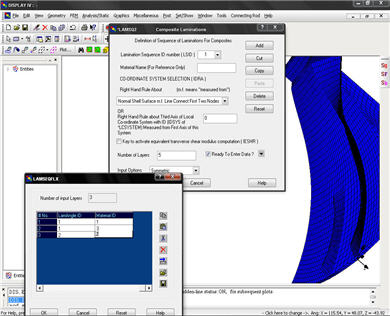NISA II/COMPOSITE is the most powerful and economical general purpose finite element program to solve a wide range of static, dynamic, buckling, heat transfer, optimization and nonlinear (both geometric and material) analysis problems encountered in aerospace, mechanical and civil engineering environments. NISA II/COMPOSITE, which is a part of NISA II, is tailored specifically for accurate and efficient analysis of composite structures using state-of-the-art solution methods, convergence techniques and time integration schemes. NISA II/COMPOSITE is considered the world's best program for solving linear and nonlinear structures made of composite materials. The COMPOSITE module is directly interfaced with DISPLAY III/IV for post-processing the results.### COMPOSITE ELEMENT LIBRARY

• 3D layered composite shell
• 3D layered sandwich shell
• 3D solid
• Axisymmetric layered composite solid

### ANALYSIS FEATURES

• No restriction on the lamination
• Variable thickness and rotation angles
• Edge effects and delamination can be predicted
• Nodal temperatures and temperature gradients may be specified
• Temperature dependent material properties
• Interlaminar stresses satisfying 3D equilibrium equations

### MODELING FEATURES

• Graphical representation of lamina thickness and angles across the laminate
• Draping of fabric over surfaces of general curvature

### FAILURE THEORIES

• Maximum stress
• Modified Hill-Mises
• Tsai-Wu
• Delamination

### OUTPUT

• Plots of original and/or deformed geometry
• Contour plots in any layer of displacement, stress components and stress resultants
• Filtered stress output
• The ratio of actual to allowable stress is computed. The output is suppressed if this ratio is below the user specified filtering stress ratio
• Stress survey plots
• This special survey plot searches for the most critical stress ratio in all layers of each element. prints an integer number, representing the most critical percentage of allowable stress on each element
• Largest magnitudes of the displacement vector
• Highest stress resultants in descending order of magnitude